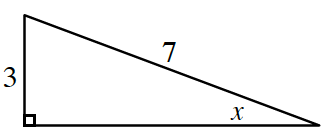### Home > PC3 > Chapter 13 > Lesson 13.2.3 > Problem13-94

13-94.

If $\sin(x)=\frac{3}{7}$ and $\sec(x)<0$, determine the exact values of $\cos\left(x\right),\tan\left(x\right),\cot\left(x\right)$, and $\sin\left(2x\right)$.

Draw a diagram. In which quadrant is $x$?Calculate the length of the missing side.

Then calculate the exact values of the other trigonometric functions.
Make sure to pay attention to the quadrant.